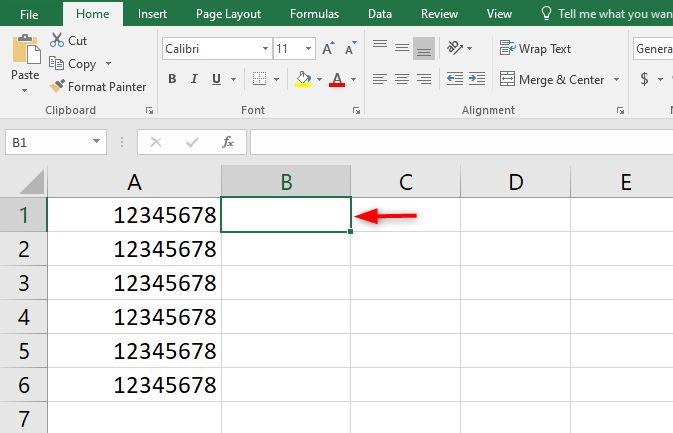# How to remove last five characters in Excel

Excel deals with both numbers and texts. You may need to remove some parts of the texts or digits from the number at some point. It is generally called " digit check," It can be a very tedious task when dealing with long strings of values.

But don't worry, excel got you covered. You can remove the last number of digits from Excel using a formula. To trigger the result, simply type the formula into the cell, and the result will be the number/text minus the last five digits. You can fill the rest of the cell by copying it down.

Stated below is the excel function for removing the last characters from a string of numbers/texts.

"LEFT(text,num_chars)"

Here is the formula in a more generalized format:

"=LEFT(A1,LEN(A1)-[number of characters to remove])"

## Remove the last five digits from a number in excel

Now, let's take a very quick formula to remove the last five digits from a number in excel. This means that a cell containing "12345678" will be trimmed to "123."

1. Firstly, open your excel spreadsheet and identify the cell you wish to work on

2. Now, identify the cell that you wish to display your result. Click the inside of the cell.Here is the formula for removing the last digits.

=LEFT(A1, LEN(A1)-1)

• A1 is the cell that contains the numbers.
• The last value, i.e -1 represents the number of values that you want to remove. You can change it according to the number of values you want to trim.
• =LEFT(A1, LEN(A1)-5) Type this formula and press enter. The last five digits will be removed from the number.3. The result will display in the next column. You can copy the cells with the formula into another cell.I hope you've seen how to remove the last digit from a number. To remove the last five digits, simply replace '1' with "5."